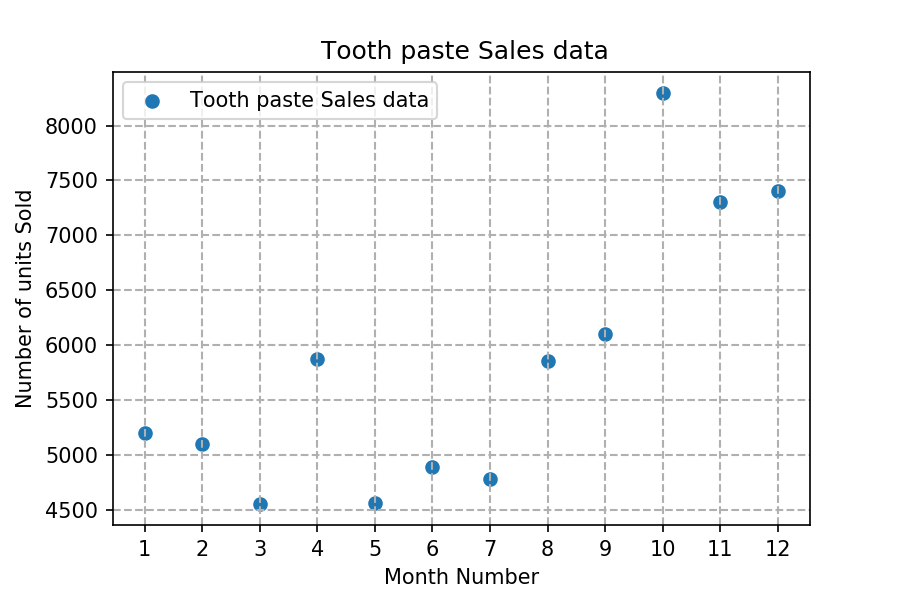Q:

# Read toothpaste sales data of each month and show it using a scatter plot

### Read toothpaste sales data of each month and show it using a scatter plot

Also, add a grid in the plot. gridline style should “–“.

The scatter plot should look like this.``````import pandas as pd
import matplotlib.pyplot as plt

monthList  = df ['month_number'].tolist()
toothPasteSalesData = df ['toothpaste'].tolist()
plt.scatter(monthList, toothPasteSalesData, label = 'Tooth paste Sales data')
plt.xlabel('Month Number')
plt.ylabel('Number of units Sold')
plt.legend(loc='upper left')
plt.title(' Tooth paste Sales data')
plt.xticks(monthList)
plt.grid(True, linewidth= 1, linestyle="--")
plt.show()``````